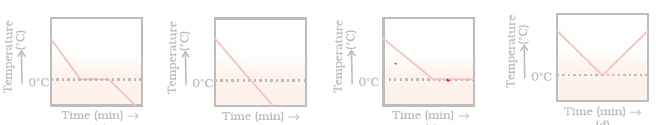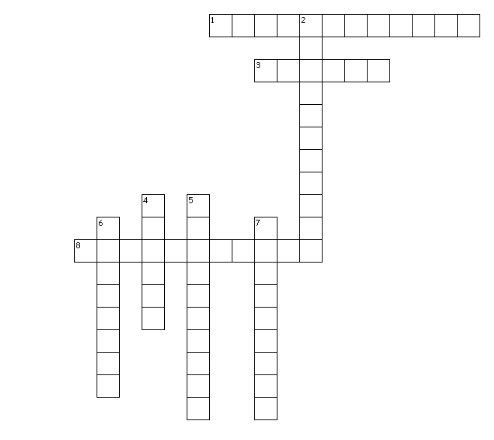# Class 9 Science CBSE Extra questions for matter in our Surroundings

Given below are the Class 9 Science CBSE Extra questions and answers for matter in our Surroundings
(c) Numericals
(e) Foundation Level Questions for Olympiads/JEE Level/PMT/KVYP
(f) Crossword
Hope you like them and do not forget to like , social share and comment at the end of the page.

Question 1.
A rubber band can change its shape on stretching. Will you classify it as solid or not? Justify?

Question 2.
Sponge, though compressible, is a solid?

Question 3.
Gases completely fill the vessel in which they are kept. Give reasons.

Question 4.
Under what conditions gases can be liquefied? In which form LPG is filled in gas cylinder?

Question 5.
What is tincture of iodine?

Question 6.
Arrange the following substances in increasing order of intermolecular force of attraction:
water, sugar, oxygen

Question 7.
Why does the temperature of a substance remain constant during melting and boiling even when heat is being supplied to it continuously?

Question 8.
Why do the gases exert more pressure on the walls of the container than the solids?

Question 9.
Why should we wear cotton clothes during summer?

Question 10.
Why does a desert cooler cool better on a hot dry day?

Question 11.
Liquid generally have lower density as compared to solids, but ice floats on water. Find out, why.

Question 12.
What is dry ice?

Question 13.
Explain why temperature remains constant during interconversion of states of matter?

Question 14.
Give reason to explain why it takes longer time to dry wet clothes in humid weather?

Question 15.
Why do people sprinkle water on the roof after a hot sunny day?

Question 16.
Write any three differences between evaporation and boiling?

Question 17.
Why does ice at 0°C appear colder than water at same temperature?

Question 18.
Why mixture does not have a fixed melting point or a fixed boiling point? Give two reasons?

Question 19.
On suffering from fever which will lower down your body temperature, more ice or ice cold water?

Question 20.
A gas exerts pressure on the walls of container, why?

Question 21.
The smell of hot sizzling food reaches you several meters away, but to get the smell from cold food you have to go close.

Question 22.
Comment upon the following: rigidity, compressibility, fluidity, filling a gas container, shape, kinetic energy, and density.

Question 23.
What is the physical state of water at:
(a) 250°C
(b) 100°C

Question 24.
Why does our palm feel cold when we put some acetone or petrol or perfume on it?

Question 25.
Give reason for the following observations.
(a) Naphthalene balls disappear with time without leaving any solid.
(b) We can get the smell of perfume sitting several meters away.

Question 26.
Give two reasons to justify-
(a)Water at room temperature is a liquid.
(b)An iron almirah is a solid at room temperature.

Question 27.
A glass tumbler containing hot water is kept in the freezer compartment of a refrigerator (temperature < 00C). If you could measure the temperature of the content of the tumbler, which of the following graphs would correctly represent the change in its temperature as a function of time.Question 28.
What is condensation? How is the condensation of a gas carried out?

Question 29.
Why do solids not diffuse?

Question 30.
Which state of matter is compressible? Why?

## Numericals

Question 31.
i.Convert the following Kelvin temperature to degrees Celsius.
a. 173 K
b. 273 K
c. 400 K
ii.Convert the following Celsius temperature to Kelvin temperature.
a. -73 ° C
b. -23 ° C
c. 100 ° C

Question 32.
Convert the following Kelvin temperature to degrees Celsius.
a. 175 K
b. 295 K
c. 300 K
d. 225 K

Question 33.
Convert the following Celsius temperature to Kelvin temperature.
a. 25 0C
b. -15 0C
c. 0 0C
d. 3 0C

Question 34.
What is the physical state of water at the following temperatures?
(a) 25 0C
(b) 0 0C
(c) 100 0C

Question 35.
The process in which a solid is converted directly into a gas is called sublimation. Iodine is an element that sublimes. A sample of solid iodine in a stoppered flask was allowed to stand undisturbed for several days. Crystals of solid iodine grew on the sides of the flask. Explain at the molecular level what happened?
Question 36.
Give three examples of crystalline and amorphous solids.

Question 37. Explain the diffusion of copper sulphate into water.
Question 38. Why is motor oil more viscous than water? Does motor oil have a greater surface tension than water.
Question 39. Describe why a drop of food coloring in a glass of water slowly becomes evenly distributed without the need for stirring?
Question 40. Liquid mix more slowly than gases. Why?
Question 41. Define the following terms:
a. Melting point
b. Freezing point
c. Boiling point

## Foundation Level Questions for Olympiads/JEE Level/PMT/KVYP

Question 42.
A constant pressure tank of gas at 1.01 Atm has propane in it at 150C when it is at 255 cubic meters. What is its volume at 480C?

Question 43.
What is the mass of 15 liters of chlorine gas at STP?

Question 44.
How many liters of hydrogen at 00C and 1400mm of Hg are produced if 15g of magnesium reacts with sulphuric acid?

Question 45.
A 250 Kg tank of liquid butane (C4H10) burns to produce carbon dioxide at 1200C. What volume of carbon dioxide is produced at 1 Atm?

Question 46.
How many liters of product at 950 mmHg and 00C is produced by the burning of three liters of acetylene (C2H2) at 5 atm and 200C?

Question 47.
A fixed quantity of gas at 230C exhibits pressure of 748 torr and occupies a volume of 10.3 L
a)Use Boyle's law to calculate the volume the gas will occupy at 230C if the pressure is increased to 1.88 atm.
b)Use Charles' law to calculate the volume the gas will occupy if the temperature is increased to 1650C while the pressure is held constant.

Question 48.
(a) Write the ideal- gas equat ion, and give the units used for each term in the equation when
Given that R = 0.0821 L-atm/mol- K
(b) What is an ideal gas?

Question 49.
A certain volume of a gas is under a pressure of 900 mm of Hg. When the pressure is increased by 300 mm, the gas occupies 2700 ml. if this change occurs at a constant temperature, calculate the initial volume of the gas.
Question 50.
At a pressure of 2 atmosphere a fixed mass of hydrogen occupies a volume of 8 liters. What pressure must be maintained if the volume is to be increased to 10 liters, temperature remaining constant?
Question 51.
A given mass of a gas occupies 960 ml at 270C C. What volume will it occupy if the temperature is raised to 1770C C, pressure remaining constant?

## Crossword Puzzle

Question 52.
Crossword PuzzleAcross
1. BEC stands for Bose-Einstein-______
3. The state consists of super energetic and super excited particles
8. Conversion of solid to vapour is called ______
Down
2. This is the phenomenon of change of a liquid into vapours at any temperature below its boiling point
4. SI unit of Temperature
5. CNG stands ____ natural gas
6. It is the amount of water vapour present in air.
7. LPG stands for ______petroleum gas.

## Summary

This matter in our surroundings class 9 extra questions with answers is prepared keeping in mind the latest syllabus of CBSE . This has been designed in a way to improve the academic performance of the students. If you find mistakes , please do provide the feedback on the mail.